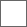• 6回复贴，共1

### 帝国知识科普贴（续更）

PS：公式自己想的，可能有点简陋，勿怪！H=Max(1,Sum(Max(0,i-j))*k)
i=单位A的i类攻击
j=Or(单位B的i类防御,单位B的默认护甲)

H=Max(1,Sum(Max(0,单位A的i类攻击-Or(单位B的i类防御,单位B的默认护甲))*k)

1.H为单位A对单位B的伤害
2.Max为最大值函数，Max(x,y)是取x,y中的最大值。
3.Sum为求和函数。
4.Or函数，二选一。
5.i为单位A的i类型攻击
6.j为单位B的i类型防御或单位B的默认护甲。
7.k为高地系数。

PS:一般版本的帝国（原版帝国，高清版，UP1.2～1.4）里高地有7种，高地1（低洼），高地2（平地），高地3～7。UP1.5又扩充了几个高地（高地8～11）

H=Max(1,(6+0+21+0+16+0)*1)=Max(1,43)=43

H=Max(1,(1+0+0+0)*1.25)=Max(1,1.25)=1.25

H=Max(1,(3+3+6+3)*1)=Max(1,15)=15https://tieba.baidu.com/p/5319370174

https://tieba.baidu.com/p/4306824282

[兵营]近卫军，精锐近卫军（16）#### 扫二维码下载贴吧客户端